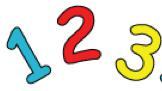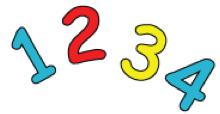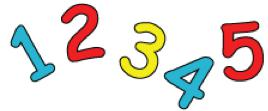#### You may also like### Pebbles

Place four pebbles on the sand in the form of a square. Keep adding as few pebbles as necessary to double the area. How many extra pebbles are added each time?### Bracelets

Investigate the different shaped bracelets you could make from 18 different spherical beads. How do they compare if you use 24 beads?### Sweets in a Box

How many different shaped boxes can you design for 36 sweets in one layer? Can you arrange the sweets so that no sweets of the same colour are next to each other in any direction?

# Curious Number

## Curious NumberCan you order the digits 1, 2 and 3 to make a number which is divisible by 3?
And when the final digit is removed again it becomes a two-digit number divisible by 2,
then finally a one-digit number divisible by 1?

Can you order the digits 1, 2, 3 and 4 to make a number which is divisible by 4?
And when the final digit is removed it becomes a three-digit number which is divisible by 3.
And when the final digit is removed again it becomes a two-digit number divisible by 2,
then finally a one-digit number divisible by 1?

Can you order the digits 1, 2, 3, 4 and 5 to make a number which is divisible by 5?
And when the final digit is removed it becomes a four-digit number which is divisible by 4.
And when the final digit is removed it becomes a three-digit number which is divisible by 3.
And when the final digit is removed again it becomes a two-digit number divisible by 2,
then finally a one-digit number divisible by 1?

What systems are you using?
What do you know about numbers which can be divided by 3, 4, 5?
Now what about taking this further for digits 1, 2, 3, 4, 5, and 6?
What do you know about numbers which can be divided by 6, 7, 8 and 9?

### Why do this problem?

This problem is intentionally difficult for Stage 2 as it stands, but this allows us to focus on promoting resilience and perseverance with children. We have purposefully written these notes so that simplified versions are tried first. This means that insights can then be applied to the problem itself and children can begin to build up their perseverance skills. The problem presents opportunities for discussing efficient or elegant solutions and working in a systematic way. Some knowledge of divisibility rules is essential.

### Possible approach

Write up the number 123 (one hundred and twenty three) on the board for all to see and give time for learners to talk together about anything they notice or are curious about. Share some suggestions, which might include, for example, that it is odd, it has three digits, the digits are consecutive, it is a multiple of three ...  There will be many ideas hopefully!

Explain that this is in fact a special number. If you look at the number created by all three digits ($123$), it is divisible by $3$. If you look at the number created by just the first two digits ($12$), it is divisible by $2$. If you look at just the first digit ($1$), it is divisible by $1$. Say something along the lines of "I wonder whether there are any other ways of arranging the digits $1$, $2$ and $3$ to create a number which has these same properties?" In other words, the challenge is to order the digits $1$, $2$ and $3$ to make a number which is divisible by $3$ ... so that when the final digit is removed it becomes a two-figure number divisible by $2$ ... and when the final digit is removed again it becomes a one-figure number divisible by $1$.

It might be worth beginning this briefly on the board so that everyone is very clear what the question means: You could suggest that another way of ordering the numbers 1, 2 and 3 is, for example, 2 then 3 then 1 to make the number two hundred and thirty one (231). So, is the number 231 divisible by 3? How do they know? [Yes, it is. Some children might mentally perform a division calculation. Others might know that if the digits add to a number that is divisible by 3, then the whole number is divisible by 3.] So far, so good. Taking off the last digit, we're left with the number 23. Is this divisible by 2? [No - it's odd.] So, the number 231 doesn't meet all the requirements. At this point, invite pairs or small groups to continue working on this problem and give them a taste of what their next challenges will be: Can they do the same for four-digit numbers using the digits 1, 2, 3 and 4? How about five-digit numbers using 1, 2, 3, 4 and 5? A six-digit number ... etc?

Once the children have had a go at the three-digit and perhaps four-digit possibilities, bring them together to talk about their methods.  This will bring up the fact that some digits have to go in certain places.  For example, which places have to have even numbers? This might also be a good opportunity to discuss other divisibility rules.   Is there a method which stands out as being particular efficient?  Or is there a method that some children particularly like for whatever reason?  (NB It is not possible to find a four-digit or a five-digit number that follow these rules but you can expect the children to be able to convince you this is the case.)  After this discussion, learners could continue to work on the problem and they may well change the method they use.

The plenary could involve sharing solutions to the six-digit problem but, in particular, invite the children to comment on how having a go at simpler versions of the task helped them tackle the six-digit (or more) challenge.  How do they think they might have got on if they'd have been presented with the six-digit version at the start?   What does this tell us about solving mathematical problems?

### Key questions

What makes a number divisible by one/two/three/four/five/six ...?
Where do the even numbers have to go?
So where do the odd numbers have to go?
Where does the five have to go?
What could you do if you are stuck?

### Possible extension

Learners could attempt to order the ten digits from 0 - 9 in the same way which is the basis of the very challenging American Billions problem.

### Possible support

The tables on this sheet might help learners organise their working - the columns can represent place value.